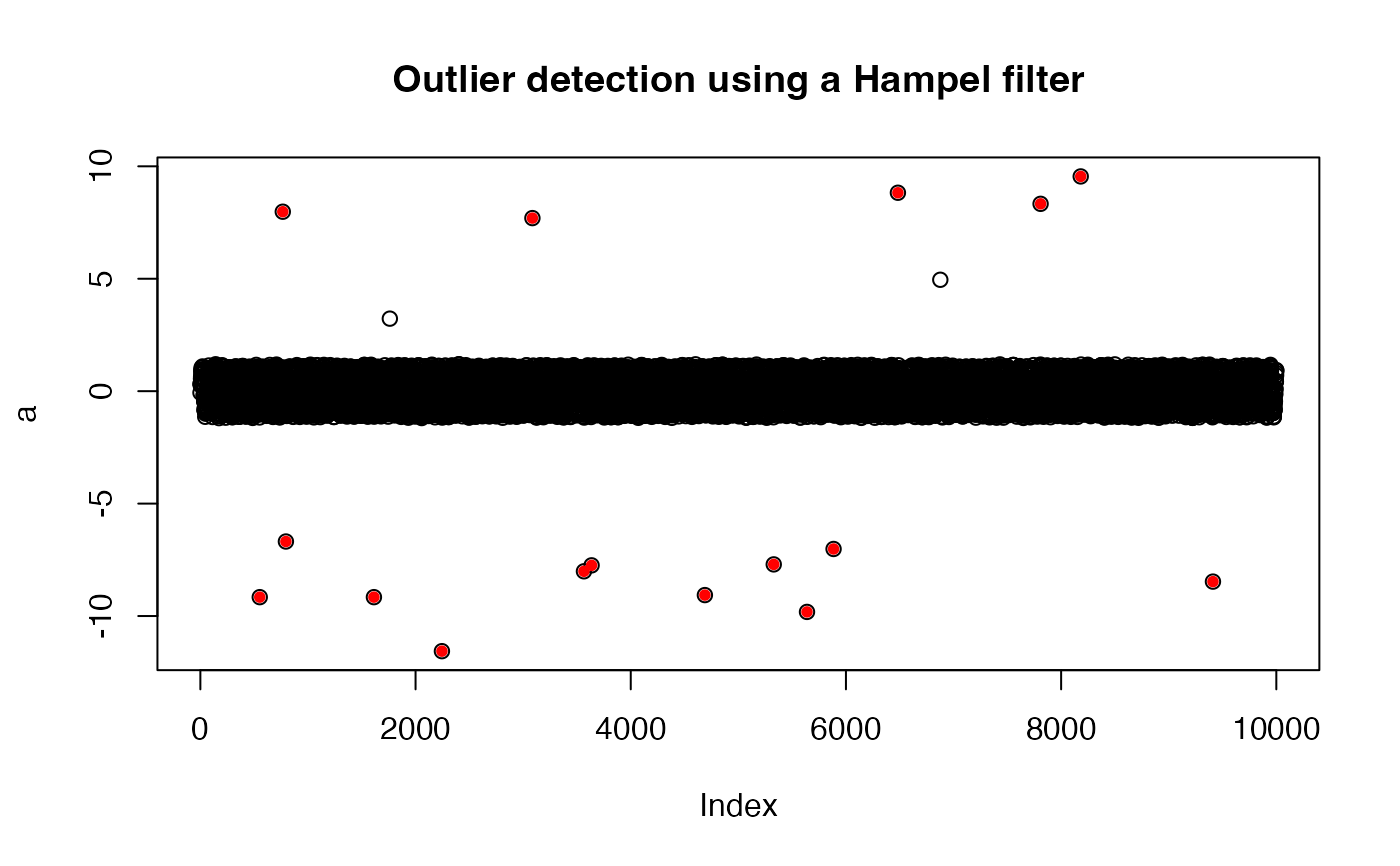A wrapper for the roll_hampel() function that counts outliers using either a user specified threshold value or a threshold value based on the statistics of the incoming data.

findOutliers(
x,
width = 25,
thresholdMin = 7,
selectivity = NA,
fixedThreshold = TRUE
)

## Arguments

x Numeric vector. Integer width of the rolling window. Numeric threshold for outlier detection Value between [0-1] used in determining outliers, or NA if fixedThreshold=TRUE. Logical specifying whether outlier detection uses selectivity (see Details).

## Value

A vector of indices associated with outliers in the incoming data x.

## Details

The thresholdMin level is similar to a sigma value for normally distributed data. Hampel filter values above 6 indicate a data value that is extremely unlikely to be part of a normal distribution (~ 1/500 million) and therefore very likely to be an outlier. By choosing a relatively large value for thresholdMin we make it less likely that we will generate false positives. False positives can include high frequency environmental noise.

With the default setting of fixedThreshold = TRUE any value above the threshold is considered an outlier and the selectivity is ignored.

The selectivity is a value between 0 and 1 and is used to generate an appropriate threshold for outlier detection based on the statistics of the incoming data. A lower value for selectivity will result in more outliers while a value closer to 1.0 will result in fewer. If fixedThreshold=TRUE, selectivity may have a value of NA.

When the user specifies fixedThreshold=FALSE, the thresholdMin and selectivity parameters work like squelch and volume on a CB radio: thresholdMin sets a noise threshold below which you don't want anything returned while selectivity adjusts the number of points defined as outliers by setting a new threshold defined by the maximum value of roll_hampel multiplied by selectivity.

width, the window width, is a parameter that is passed to roll_hampel().

## Note

This function is copied from the seismicRoll package.

roll_hampel

## Examples

# Noisy sinusoid with outliers
a <- jitter(sin(0.1*seq(1e4)),amount=0.2)
indices <- sample(seq(1e4),20)
a[indices] <- a[indices]*10

# Outlier detection should identify many of these altered indices
sort(indices)
#>    552  766  795 1613 1761 2167 2245 2794 3086 3565 3636 4689 5329 5638 5885
#>  6483 6877 7809 8182 9411o_indices <- findOutliers(a)
o_indices
#>    552  766  795 1613 2245 3086 3565 3636 4689 5329 5638 5885 6483 7809 8182
#>  9411
plot(a)
points(o_indices, a[o_indices], pch = 16, cex = 0.8, col = 'red')
title("Outlier detection using a Hampel filter")